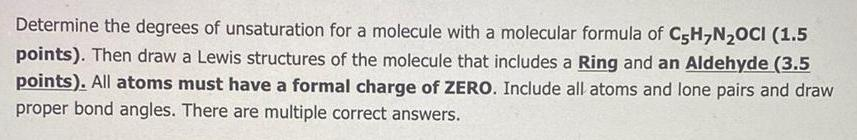Organic Chemistry
Hydrocarbons
Determine the degrees of unsaturation for a molecule with a molecular formula of C5H7N₂OCI (1.5 points). Then draw a Lewis structures of the molecule that includes a Ring and an Aldehyde (3.5 points). All atoms must have a formal charge of ZERO. Include all atoms and lone pairs and draw proper bond angles. There are multiple correct answers.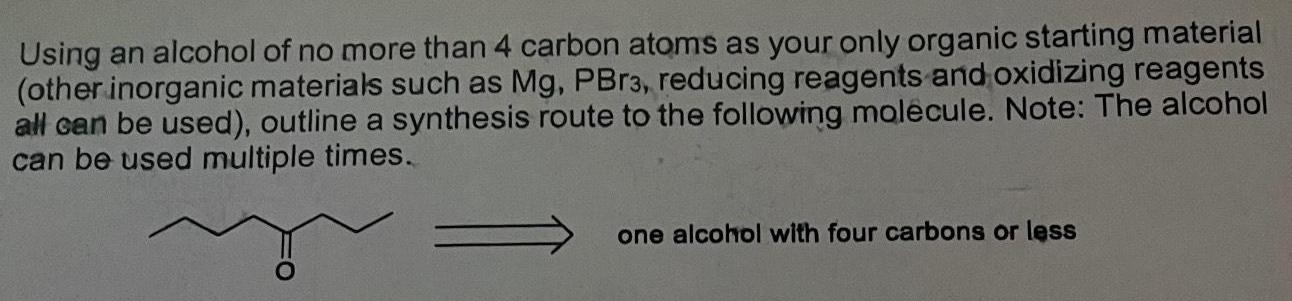Organic Chemistry
Hydrocarbons
Using an alcohol of no more than 4 carbon atoms as your only organic starting material (other inorganic materials such as Mg, PBr3, reducing reagents and oxidizing reagents all can be used), outline a synthesis route to the following molecule. Note: The alcohol can be used multiple times.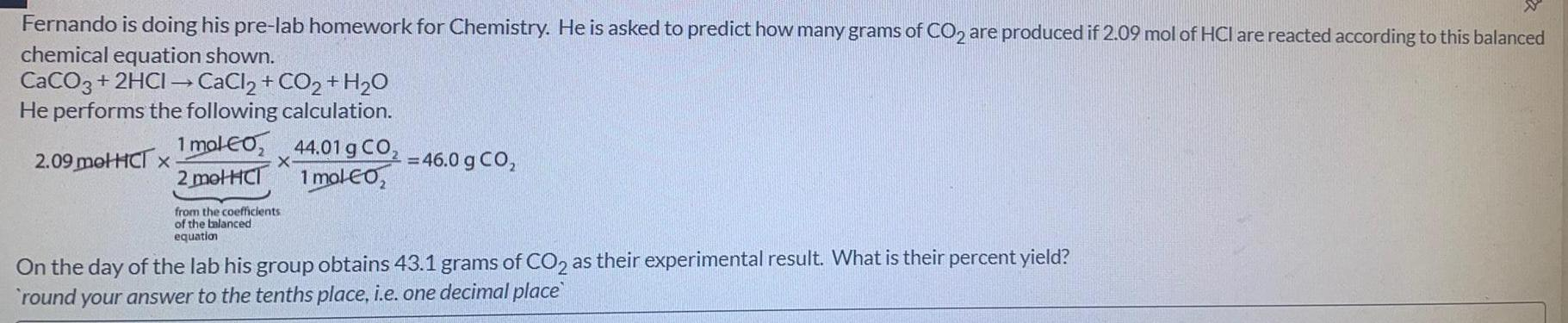Organic Chemistry
Hydrocarbons
Fernando is doing his pre-lab homework for Chemistry. He is asked to predict how many grams of CO2 are produced if 2.09 mol of HCI are reacted according to this balanced chemical equation shown. CaCO3 + 2HCI → CaCl2 + CO2 + H2₂O He performs the following calculation. 1 mol CO₂ 2 metHCI 2.09 metHCT x X from the coefficients of the balanced equation 44.01 g CO₂ 1 mol CO₂ = 46.0 g CO₂ On the day of the lab his group obtains 43.1 grams of CO2 as their experimental result. What is their percent yield? round your answer to the tenths place, i.e. one decimal place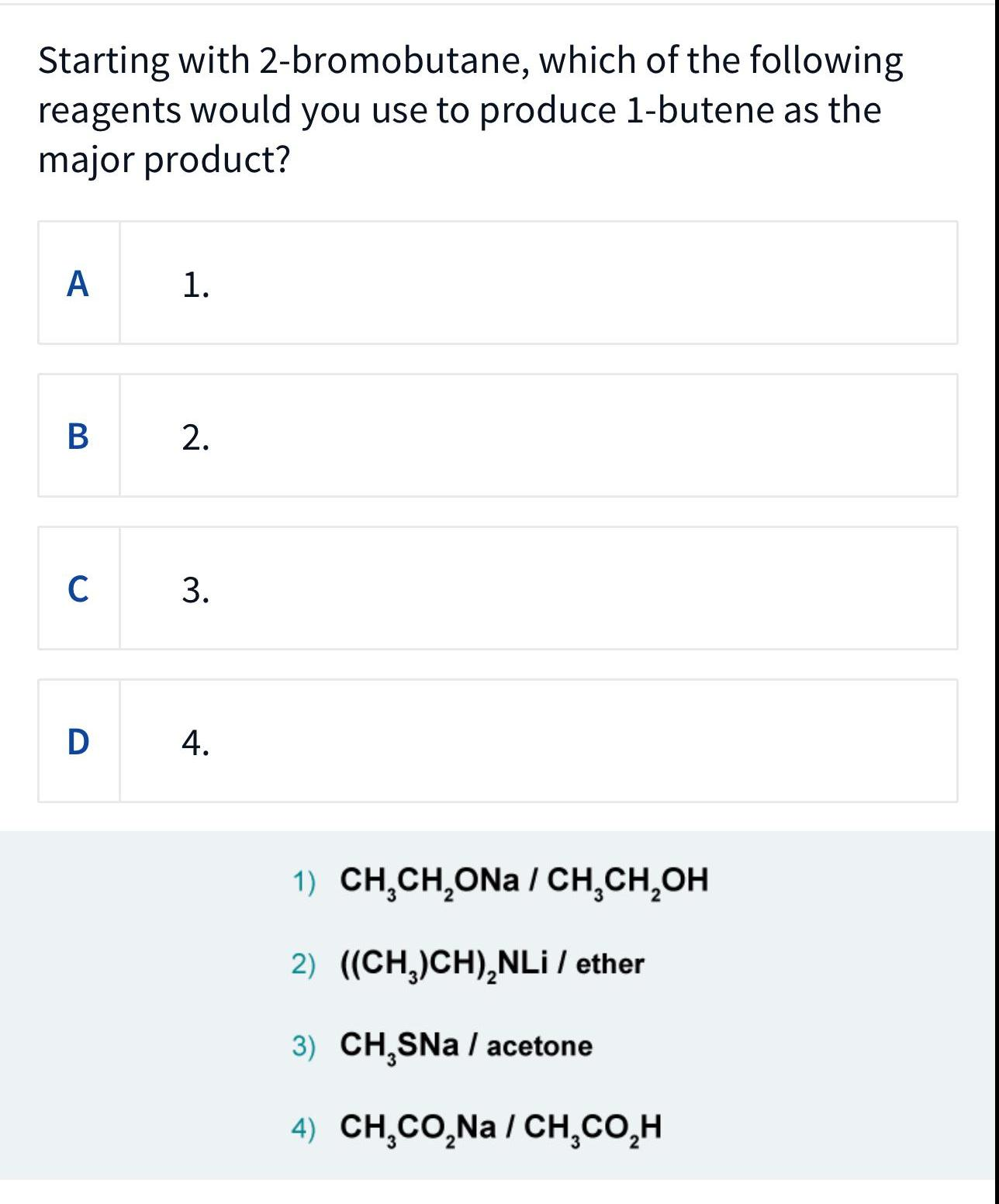Organic Chemistry
Hydrocarbons
Starting with 2-bromobutane, which of the following reagents would you use to produce 1-butene as the major product? 1. 2. 3. 4. 1) CH₂CH₂ONA / CH₂CH₂OH 2) ((CH₂)CH)₂NLi / ether 3) CH₂SNa / acetone 4) CH₂CO₂Na/CH₂CO₂H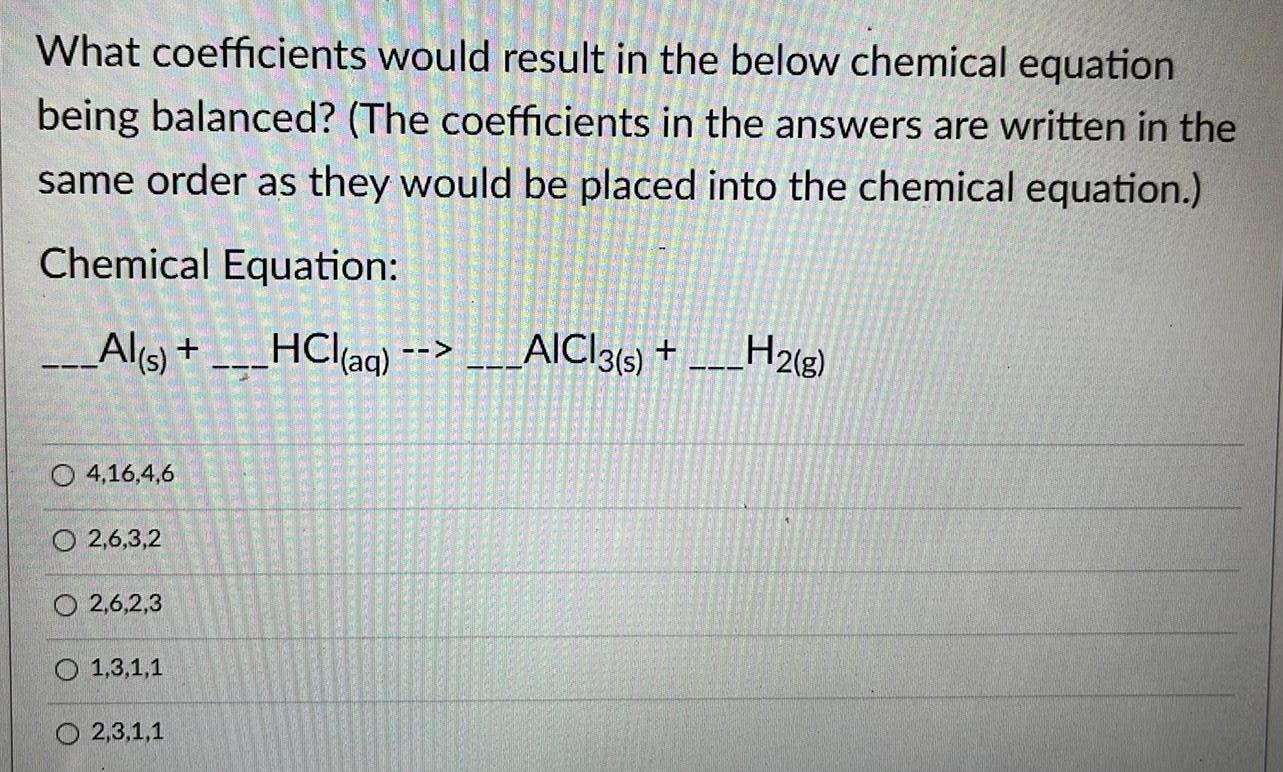Organic Chemistry
Hydrocarbons
What coefficients would result in the below chemical equation being balanced? (The coefficients in the answers are written in the same order as they would be placed into the chemical equation.) Chemical Equation: _Al(s) + HCl(aq) --> ___AICI3(s) +___H2(g) 4,16,4,6 2,6,3,2 2,6,2,3 1,3,1,1 O2,3,1,1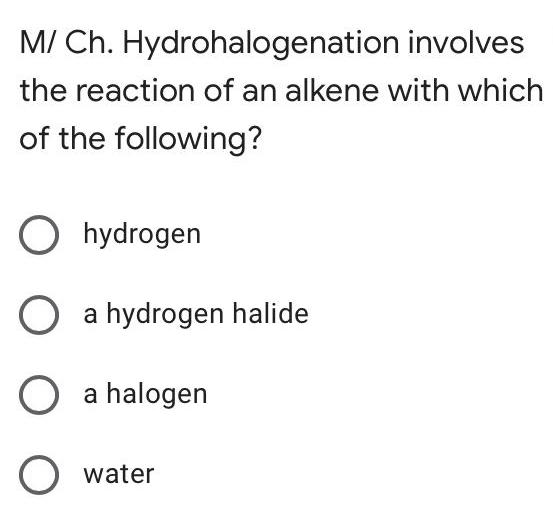Organic Chemistry
Hydrocarbons
M/ Ch. Hydrohalogenation involves the reaction of an alkene with which of the following? hydrogen a hydrogen halide a halogen water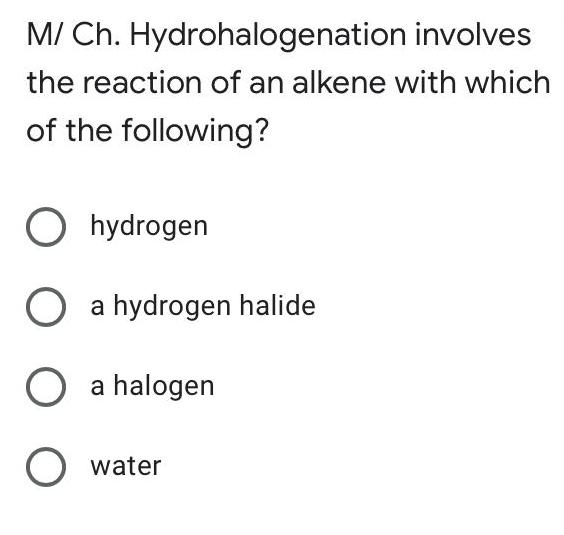Organic Chemistry
Hydrocarbons
M/ Ch. Hydrohalogenation involves the reaction of an alkene with which of the following? hydrogen a hydrogen halide a halogen water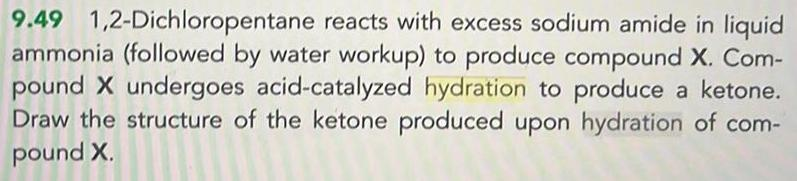Organic Chemistry
Hydrocarbons
1,2-Dichloropentane reacts with excess sodium amide in liquid ammonia (followed by water workup) to produce compound X. Com- pound X undergoes acid-catalyzed hydration to produce a ketone. Draw the structure of the ketone produced upon hydration of com- pound X.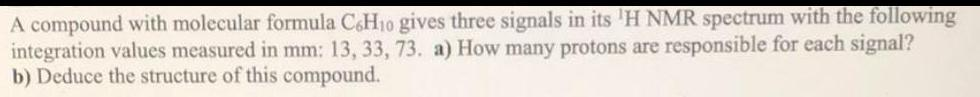Organic Chemistry
Hydrocarbons
A compound with molecular formula C6H₁0 gives three signals in its 1H NMR spectrum with the following integration values measured in mm: 13, 33, 73. a) How many protons are responsible for each signal? b) Deduce the structure of this compound.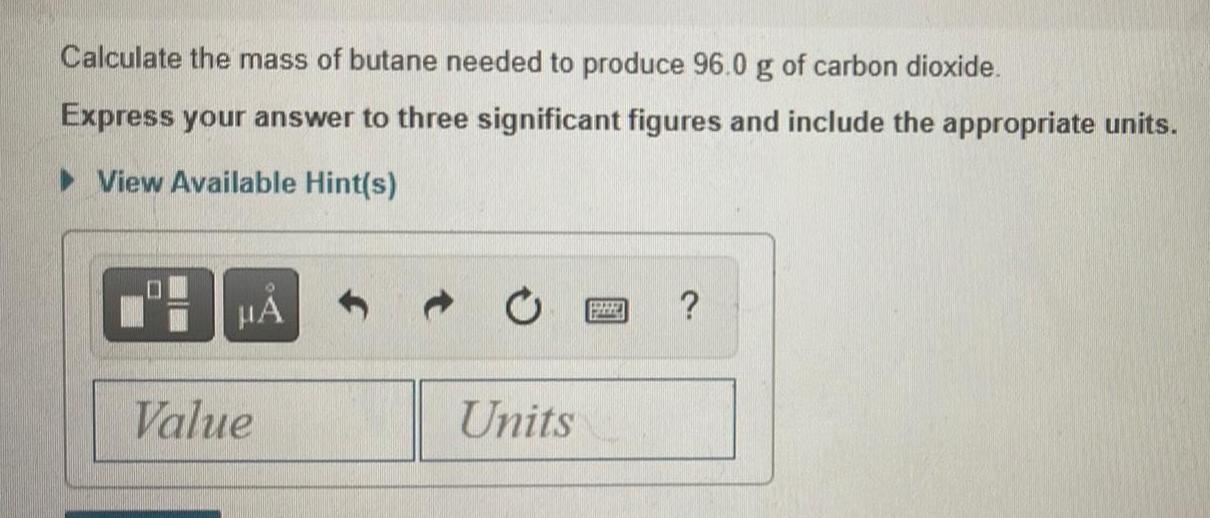Organic Chemistry
Hydrocarbons
Calculate the mass of butane needed to produce 96.0 g of carbon dioxide. Express your answer to three significant figures and include the appropriate units.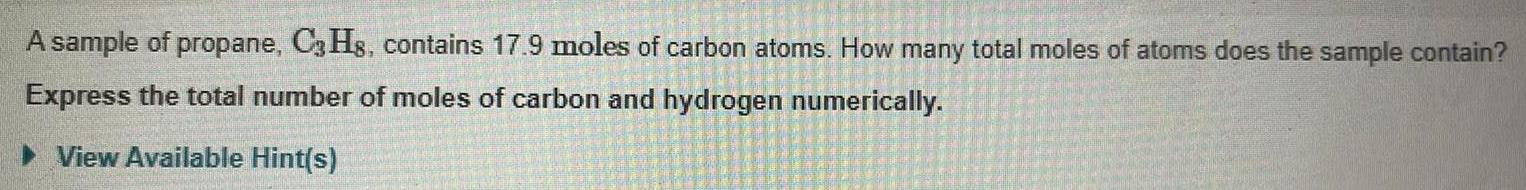Organic Chemistry
Hydrocarbons
A sample of propane, C3H8, contains 17.9 moles of carbon atoms. How many total moles of atoms does the sample contain? Express the total number of moles of carbon and hydrogen numerically.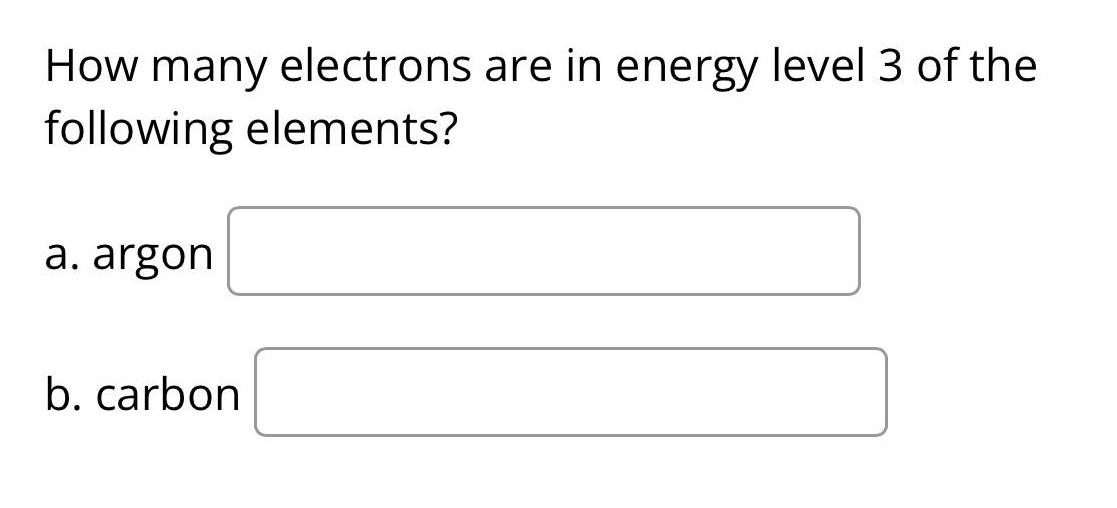Organic Chemistry
Hydrocarbons
How many electrons are in energy level 3 of the following elements? a. argon b. carbon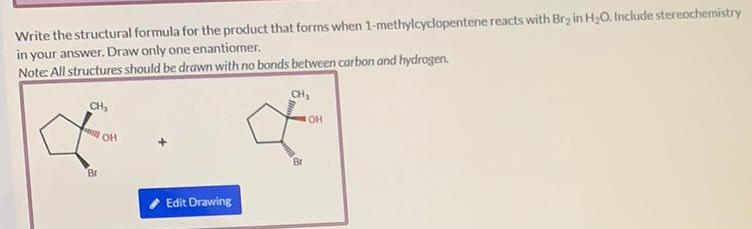Organic Chemistry
Hydrocarbons
Write the structural formula for the product that forms when 1-methylcyclopentene reacts with Br₂ in H₂O. Include stereochemistry in your answer. Draw only one enantiomer. Note: All structures should be drawn with no bonds between carbon and hydrogen.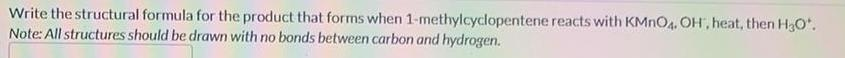Organic Chemistry
Hydrocarbons
Write the structural formula for the product that forms when 1-methylcyclopentene reacts with KMnO4. OH, heat, then H₂O*. Note: All structures should be drawn with no bonds between carbon and hydrogen.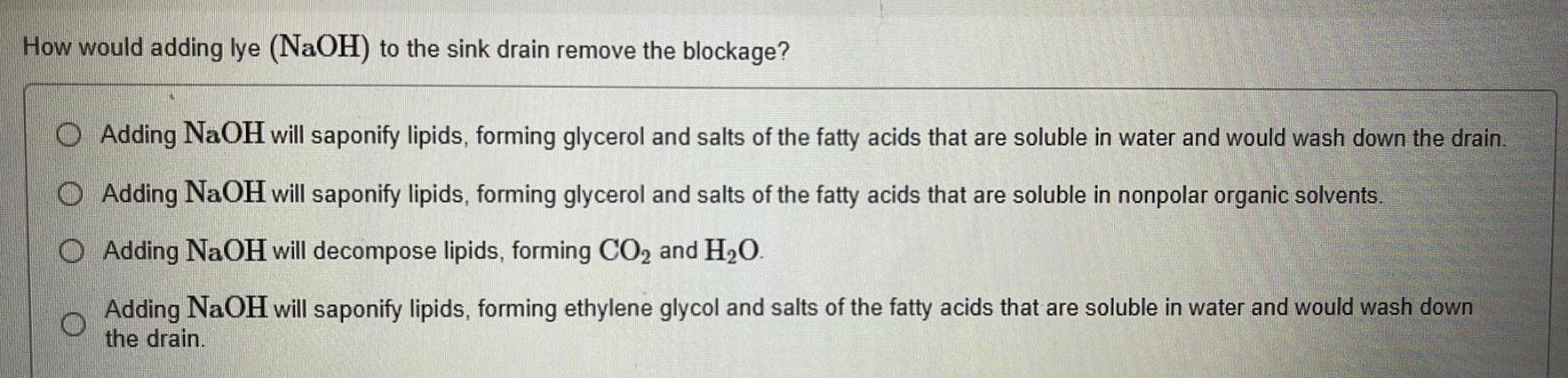Organic Chemistry
Hydrocarbons
How would adding lye (NaOH) to the sink drain remove the blockage? Adding NaOH will saponify lipids, forming glycerol and salts of the fatty acids that are soluble in water and would wash down the drain. Adding NaOH will saponify lipids, forming glycerol and salts of the fatty acids that are soluble in nonpolar organic solvents. Adding NaOH will decompose lipids, forming CO₂ and H₂O. Adding NaOH will saponify lipids, forming ethylene glycol and salts of the fatty acids that are soluble in water and would wash down the drain.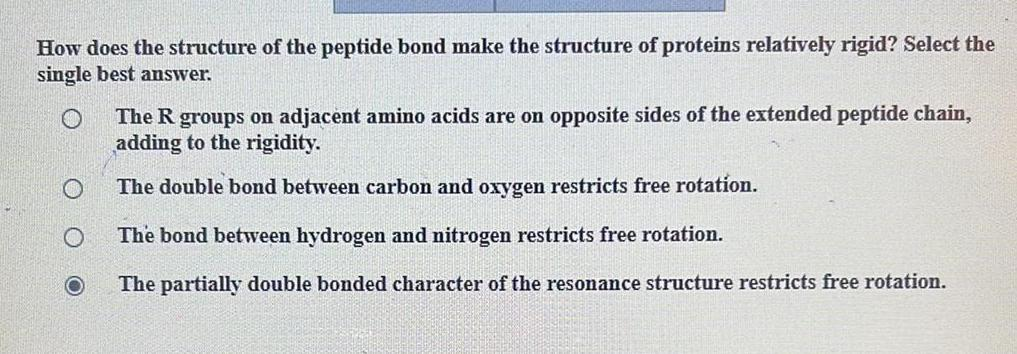Organic Chemistry
Hydrocarbons
How does the structure of the peptide bond make the structure of proteins relatively rigid? Select the single best answer. The R groups on adjacent amino acids are on opposite sides of the extended peptide chain, adding to the rigidity. The double bond between carbon and oxygen restricts free rotation. The bond between hydrogen and nitrogen restricts free rotation. The partially double bonded character of the resonance structure restricts free rotation.